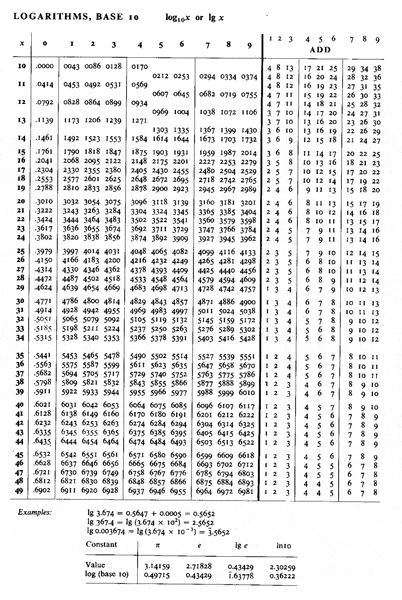# Relation between units of attenuation

ParoxysmX

## Homework Statement

It is customary to express fibre loss in units of dB/km:

$\alpha(dB/km) = \frac{10log(P_{in}/P_{out})}{L(km)}$

where $P_{in}$ is the power entering the fibre and $P_{out}$ is the power leaving the fibre. Show that $\alpha(dB/km)$ = 4343$\alpha$($m^{-1}$)

## The Attempt at a Solution

Dividing through by decibels -should- give attenuation per kilometre, then converting to metres would give the right dimension, but how can you come up with a coefficient without any numbers to work with? Also, is attenuation per metre just an arbitrary value of loss per metre?

## Answers and Replies

Homework Helper
Hi ParoxysmX!Dividing through by decibels -should- give attenuation per kilometre, then converting to metres would give the right dimension, but how can you come up with a coefficient without any numbers to work with?

I don't understand your question.Does it help if I point out that Pout/Pin is a dimensionless number, and therefore so is log(Pout/Pin) ?
Also, is attenuation per metre just an arbitrary value of loss per metre?

I think the idea behind it is that if the attenuation is constant, that is the same as saying that the power "does not remember its history" …

P(x)= ekx,

so P(L+x) = P(L)P(x) …
the power loss over distance x is always P(x), no matter at what distance L you startHomework Helper
Gold Member
I think there might be some confusion with the notation. I believe that what you want to show is that

if ##P_{out} = P_{in}e^{-\lambda L}##, then ##\alpha(dB/km) = 4343\lambda(m^{-1})##.

##L## is measured in meters in the exponential expression so that ##\lambda## has the units of m-1.

ParoxysmX
I think there might be some confusion with the notation. I believe that what you want to show is that

if ##P_{out} = P_{in}e^{-\lambda L}##, then ##\alpha(dB/km) = 4343\lambda(m^{-1})##.

##L## is measured in meters in the exponential expression so that ##\lambda## has the units of m-1.

Where does $\lambda$ come into it? Is that simply the wavelength of the light entering the fibre? My question sheet definitely says ##\alpha(dB/km) = 4343\alpha(m^{-1})##.

e; I see that ##P_{out} = P_{in}e^{-\lambda L}## is the same as the version we were taught where ##P_{out} = P_{in}e^{-\alpha L}##. But then, I'm still not sure where 4343 comes from without having data to work with.

Last edited:
Homework Helper
Gold Member
##\lambda## is just the notation I picked for the coefficient of ##L## in the argument of the exponential. Apparently, your question sheet uses the same notation ##\alpha## for two different quantities. I find this a little confusing, but I guess it is standard practice. Including the units is then essential in order to distinquish them.

Last edited:
ParoxysmX
Yeah ok, so this essentially comes down to a conversion between bases. ##\αlpha(dB/km)## should equal ##2303 \αlpha(m−1)## if you account for the difference between metres and kilometres, shouldn't it?

Homework Helper
Gold Member
In your formula for ##\alpha(dB/km)## you need to know what base you are using for the log. I suspect it is base 10. Likewise, for ##\alpha(m^{-1})## you need to know if it is defined using ##P_{out} = P_{in}e^{-\alpha L}## or ##P_{out} = P_{in}10^{-\alpha L}##. I suspect it is the first way.

Homework Helper
… I'm still not sure where 4343 comes from …

log10e = 0.4343

(so 100.4343 = e)

(and if you look at the top line of your log tables, you'll see it starts 0043 0086 etc, which is short for 004343 …… oooh, do people still have log tables?see)

ParoxysmX
log10e = 0.4343

(so 100.4343 = e)

(and if you look at the top line of your log tables, you'll see it starts 0043 0086 etc, which is short for 004343 …… oooh, do people still have log tables?see)

Ah yes this makes sense, thanks tiny-tim!